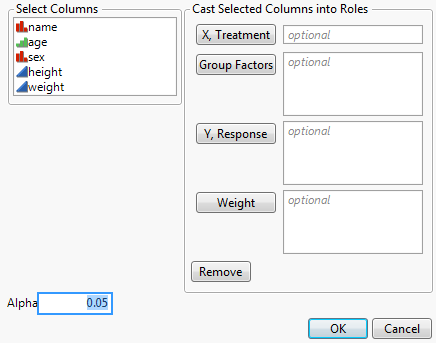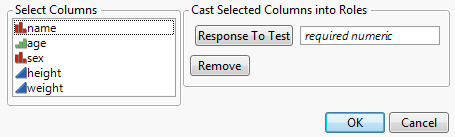Scripting Guide > Display Trees > Modal Windows > Construct a Column Dialog
Publication date: 04/12/2021

## Construct a Column Dialog

Column Dialog(), a variant of the deprecated Dialog() function, lets you prompt for column selections from the current data table or contextual data table. The OK, Cancel, and Remove buttons and the list of columns to choose from the data table are added automatically.

`dt = Open( "\$SAMPLE_DATA/Big Class.jmp" );`
`dlg = Column Dialog(`
`	Col ID = Col List( "X, Treatment", Max Col( 1 ) ),`
`	Group = Col List( "Group Factors" ),`
`	Split = Col List( "Y, Response" ),`
`	w = Col List( "Weight" ),`
`	H List( "Alpha", alpha = Edit Number( .05 ) )`
`);`

Figure 11.36 Column DialogThe following example returns a list similar to this one, depending on the user’s choices:

{Col ID = {}, Group = {}, Split = {}, w = {}, alpha = 0.05, Button( -1 )}

For each destination list, a Col List clause must be a direct argument of Column Dialog() (not nested inside some other argument). An optional MaxCol(n) argument restricts the number of data columns that can be chosen to n. Lists are always returned, although they can sometimes be empty lists. You can include as many as twelve Col List clauses.

Other items permitted in the deprecated Dialog() are permitted in Column Dialog() and have the same functionality. You can specify the minimum and maximum number of columns that are allowed in a column dialog box with the MinCol and MaxCol arguments.

You can also specify the modeling type of the columns that are allowed to be selected (Ordinal, Nominal or Continuous). You can set the width of the list using Select List Width(pixels) argument. To set the width of the column list, use Width(pixels) inside the Col List() function.

The following example generates a column dialog box that only allows the selection of exactly one numeric column:

`dt = Open( "\$SAMPLE_DATA/Big Class.jmp" );`
`rt_dlg = Column Dialog(`
`	cv = Col List( "Response To Test", MaxCol( 1 ), MinCol( 1 ), DataType( "Numeric" ) )`
`);`

Figure 11.37 Restricting Selection of ColumnsThe Data Type choices are Numeric, Character, and Any.

In addition, use the Columns specification to pre-fill some column selections. For example, the following script assigns height to the X role and weight and age to the Y role:

`dt = Open( "\$SAMPLE_DATA/Big Class.jmp" );`
`dlg = Column Dialog(`
`	xCols = Col List( "X, Factors", Columns( :height ) ),`
`	yCols = Col List( "Y, Response", Columns( :weight, :age ) )`
`);`
Want more information? Have questions? Get answers in the JMP User Community (community.jmp.com).